# Augment Bounding Boxes for Object Detection

This example shows how to use MATLAB®, Computer Vision Toolbox™, and Image Processing Toolbox™ to perform common kinds of image and bounding box augmentation as part of object detection workflows.

Object detector training data consists of images and associated bounding box labels. When you augment training data, you must apply identical transformations to the image and associated bounding boxes. This example demonstrates three common types of transformations:

The example then shows how to apply augmentation to training data in datastores using a combination of multiple types of transformations.

You can use augmented training data to train a network. For an example showing how to train an object detection network, see Object Detection Using Faster R-CNN Deep Learning (Computer Vision Toolbox).

Read and display a sample image and bounding box. To compare the effects of the different types of augmentation, each transformation in this example uses the same input image and bounding box.

```filenameImage = 'kobi.png'; I = imread(filenameImage); bbox = [4 156 1212 830]; label = "dog";```

Display the image and bounding box.

```annotatedImage = insertShape(I,"rectangle",bbox,"LineWidth",8); imshow(annotatedImage) title('Original Image and Bounding Box')```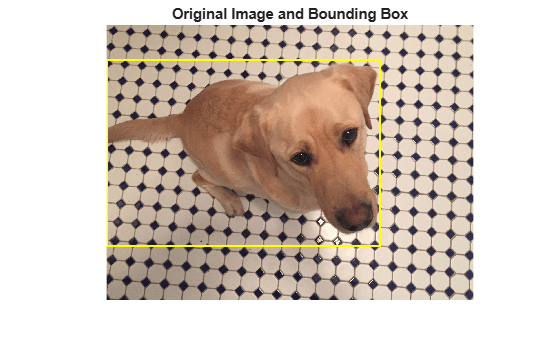### Resize Image and Bounding Box

Use `imresize` to scale down the image by a factor of 2.

```scale = 1/2; J = imresize(I,scale);```

Use `bboxresize` to apply the same scaling to the associated bounding box.

`bboxResized = bboxresize(bbox,scale);`

Display the resized image and bounding box.

```annotatedImage = insertShape(J,"rectangle",bboxResized,"LineWidth",8); imshow(annotatedImage) title('Resized Image and Bounding Box')```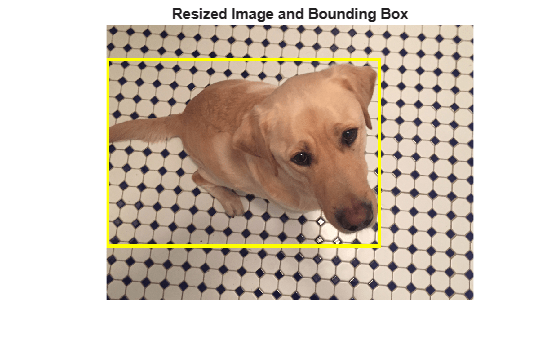### Crop Image and Bounding Box

Cropping is a common preprocessing step to make the data match the input size of the network. To create output images of a desired size, first specify the size and position of the crop window by using the `randomCropWindow2d` (Image Processing Toolbox) and `centerCropWindow2d` (Image Processing Toolbox) functions. Make sure you select a cropping window that includes the desired content in the image. Then, crop the image and pixel label image to the same window by using `imcrop`.

Specify the desired size of the cropped region as a two-element vector of the form [height, width].

`targetSize = [1024 1024];`

Crop the image to the target size from the center of the image by using `imcrop`.

```win = centerCropWindow2d(size(I),targetSize); J = imcrop(I,win);```

Crop the bounding boxes using the same crop window by using `bboxcrop`. Specify `OverlapThreshold` as a value less than 1 so that the function clips the bounding boxes to the crop window instead of discarding them when the crop window does not completely enclose the bounding box. The overlap threshold enables you to control the amount of clipping that is tolerable for objects in your images. For example, clipping more than half a person is not useful for training a person detector, whereas clipping half a vehicle might be tolerable.

`[bboxCropped,valid] = bboxcrop(bbox,win,"OverlapThreshold",0.7);`

Keep labels that are inside the cropping window.

`label = label(valid);`

Display the cropped image and bounding box.

```annotatedImage = insertShape(J,"rectangle",bboxCropped,"LineWidth",8); imshow(annotatedImage) title('Cropped Image and Bounding Box')```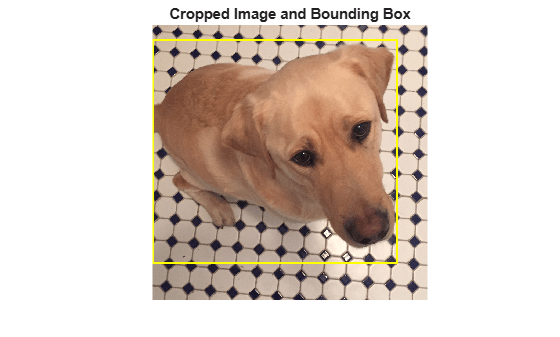### Crop and Resize Image and Bounding Box

Cropping and resizing are often performed together. You can use `bboxcrop` and `bboxresize` in series to implement the commonly used "crop and resize" transformation.

Create a crop window from a random position in the image. Crop the image and bounding box to the same crop window.

```cropSize = [1024 1024]; win = randomCropWindow2d(size(I),cropSize); J = imcrop(I,win); croppedBox = bboxcrop(bbox,win,"OverlapThreshold",0.5);```

Resize the image and box to a target size.

```targetSize = [512 512]; J = imresize(J,targetSize); croppedAndResizedBox = bboxresize(croppedBox,targetSize./cropSize);```

Display the cropped and resized image and bounding box.

```annotatedImage = insertShape(J,"rectangle",croppedAndResizedBox,"LineWidth",8); imshow(annotatedImage) title('Crop and Resized Image and Bounding Box')```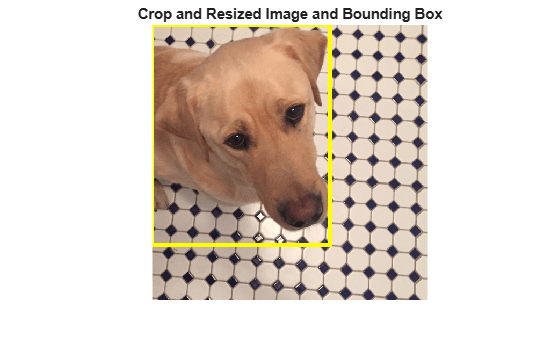### Warp Image and Bounding Box

The `randomAffine2d` (Image Processing Toolbox) function creates a randomized 2-D affine transformation from a combination of rotation, translation, scaling (resizing), reflection, and shearing. Warp an image by using `imwarp` (Image Processing Toolbox). Warp bounding boxes by using `bboxwarp`. Control the spatial bounds and resolution of the warped output by using the `affineOutputView` (Image Processing Toolbox) function.

This example demonstrates two of the randomized affine transformations: scaling and rotation.

#### Random Scale

Create a scale transformation that resizes the input image and bounding box using a scale factor selected randomly from the range [1.5,1.8]. This transformation applies the same scale factor in the horizontal and vertical directions.

`tform = randomAffine2d("Scale",[1.5 1.8]);`

Create an output view for the affine transform.

`rout = affineOutputView(size(I),tform);`

Rotate the image using `imwarp` and rotate the bounding box using `bboxwarp`. Specify an `OverlapThreshold` value of 0.5.

```J = imwarp(I,tform,"OutputView",rout); bboxScaled = bboxwarp(bbox,tform,rout,"OverlapThreshold",0.5);```

Display the scaled image and bounding box.

```annotatedImage = insertShape(J,"rectangle",bboxScaled,"LineWidth",8); imshow(annotatedImage) title('Scaled Image and Bounding Box')```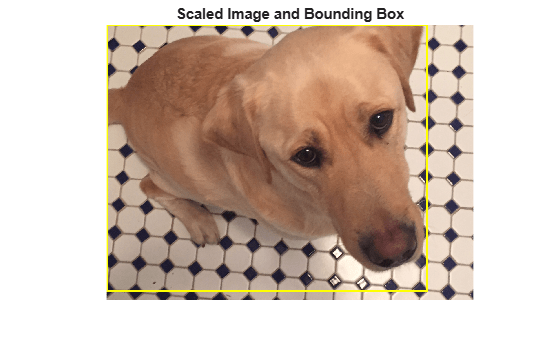#### Random Rotation

Create a randomized rotation transformation that rotates the image and box labels by an angle selected randomly from the range [-15,15] degrees.

`tform = randomAffine2d("Rotation",[-15 15]);`

Create an output view for `imwarp` and `bboxwarp`.

`rout = affineOutputView(size(I),tform);`

Rotate the image using `imwarp` and rotate the bounding box using `bboxwarp`. Specify an `OverlapThreshold` value of 0.5.

```J = imwarp(I,tform,"OutputView",rout); bboxRotated = bboxwarp(bbox,tform,rout,"OverlapThreshold",0.5);```

Display the cropped image and bounding box. Note that the bounding box returned by `bboxwarp` is always aligned to the image axes. The size and aspect ratio of the bounding box changes to accommodate the rotated object.

```annotatedImage = insertShape(J,"rectangle",bboxRotated,"LineWidth",8); imshow(annotatedImage) title('Rotated Image and Bounding Box')```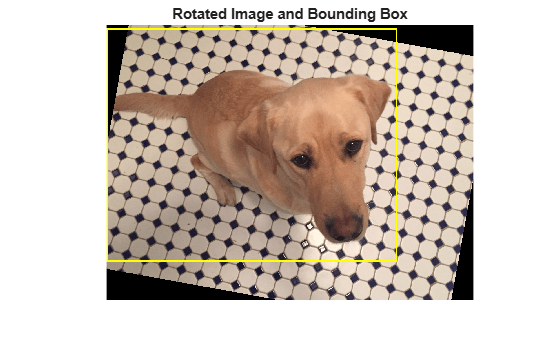### Apply Augmentation to Training Data in Datastores

Datastores are a convenient way to read and augment collections of data. Create a datastore that stores image and bounding box data, and augment the data using a series of multiple operations.

#### Create Datastores Containing Image and Bounding Box Data

To increase the size of the sample datastores, replicate the file names of the image and the bounding box and labels.

```numObservations = 4; images = repelem({filenameImage},numObservations,1); bboxes = repelem({bbox},numObservations,1); labels = repelem({label},numObservations,1);```

Create an `imageDatastore` from the training image files. Combine the bounding box and label data in a table, then create a `boxLabelDatastore` from the table.

```imds = imageDatastore(images); tbl = table(bboxes,labels); blds = boxLabelDatastore(tbl);```

Associate the image and box label pairs by combining the image datastore and box label datastore.

`trainingData = combine(imds,blds);`

Read the first image and its associated box label from the combined datastore.

```data = read(trainingData); I = data{1}; bboxes = data{2}; labels = data{3};```

Display the image and box label data.

```annotatedImage = insertObjectAnnotation(I,'rectangle',bbox,labels, ... 'LineWidth',8,'FontSize',40); imshow(annotatedImage)```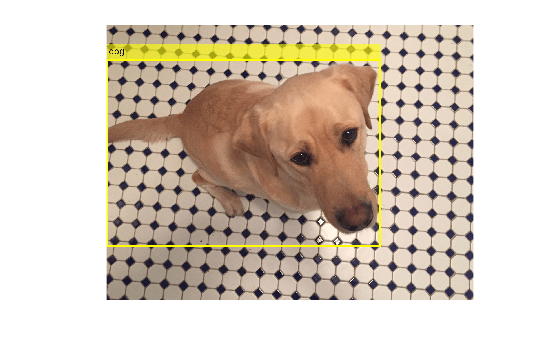#### Apply Data Augmentation

Apply data augmentation to the training data by using the `transform` function. This example performs two separate augmentations to the training data.

The first augmentation jitters the color of the image and then performs identical random horizontal reflection and rotation on the image and box label pairs. These operations are defined in the `jitterImageColorAndWarp` helper function at the end of this example.

`augmentedTrainingData = transform(trainingData,@jitterImageColorAndWarp);`

`data = readall(augmentedTrainingData);`

Display the augmented image and box label data.

```rgb = cell(numObservations,1); for k = 1:numObservations I = data{k,1}; bbox = data{k,2}; labels = data{k,3}; rgb{k} = insertObjectAnnotation(I,'rectangle',bbox,labels,'LineWidth',8,'FontSize',40); end montage(rgb)```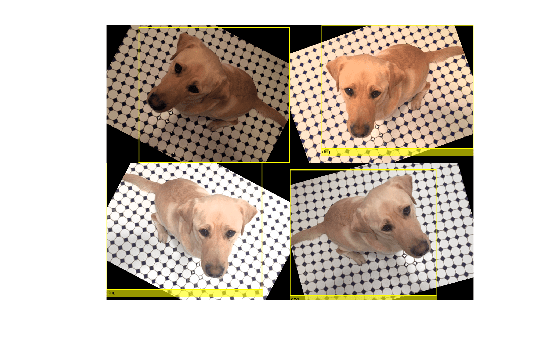The second augmentation rescales the image and box label to a target size. These operations are defined in the `resizeImageAndLabel` helper function at the end of this example.

```targetSize = [300 300]; preprocessedTrainingData = transform(augmentedTrainingData,... @(data)resizeImageAndLabel(data,targetSize));```

Read all of the preprocessed data.

`data = readall(preprocessedTrainingData);`

Display the preprocessed image and box label data.

```rgb = cell(numObservations,1); for k = 1:numObservations I = data{k,1}; bbox = data{k,2}; labels = data{k,3}; rgb{k} = insertObjectAnnotation(I,'rectangle',bbox,labels, ... 'LineWidth',8,'FontSize',15); end montage(rgb)```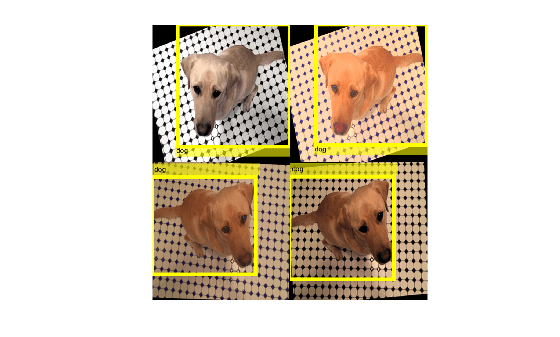#### Helper Functions for Augmentation

The `jitterImageColorAndWarp` helper function applies random color jitter to the image data, then applies an identical affine transformation to the image and box label data. The transformation consists of random horizontal reflection and rotation. The input `data` and output `out` are two-element cell arrays, where the first element is the image data and the second element is the box label data.

```function out = jitterImageColorAndWarp(data) % Unpack original data. I = data{1}; boxes = data{2}; labels = data{3}; % Apply random color jitter. I = jitterColorHSV(I,"Brightness",0.3,"Contrast",0.4,"Saturation",0.2); % Define random affine transform. tform = randomAffine2d("XReflection",true,'Rotation',[-30 30]); rout = affineOutputView(size(I),tform); % Transform image and bounding box labels. augmentedImage = imwarp(I,tform,"OutputView",rout); [augmentedBoxes, valid] = bboxwarp(boxes,tform,rout,'OverlapThreshold',0.4); augmentedLabels = labels(valid); % Return augmented data. out = {augmentedImage,augmentedBoxes,augmentedLabels}; end```

The `resizeImageAndLabel` helper function calculates the scale factor for the image to match a target size, then resizes the image using `imresize` and the box label using `bboxresize`. The input and output data are two-element cell arrays, where the first element is the image data and the second element is the box label data.

```function data = resizeImageAndLabel(data,targetSize) scale = targetSize./size(data{1},[1 2]); data{1} = imresize(data{1},targetSize); data{2} = bboxresize(data{2},scale); end```

(Computer Vision Toolbox) | (Computer Vision Toolbox) | (Computer Vision Toolbox) | (Image Processing Toolbox) | (Image Processing Toolbox) | (Image Processing Toolbox) | (Image Processing Toolbox)